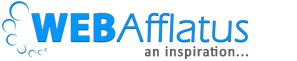# Assumptions of Linear Regression by TopBullets.comIn my previous post I mentioned some techniques one should know if they want to pursue a career in Data Analytics. In those lines, I would like to discuss a few topics in detail. This blog post is on Linear Regression, that would serve as an easy starter and then I would slowly move to other techniques. Linear regression is the most used technique in data science. Generally, when we say linear regression we refer to additive model which means dependent variable will be a function of all independent variables and there would linear and additive relationships.

Assuming you have some basic knowledge about linear regression, let’s talk about the assumptions of it and why they are important.
As mentioned earlier the basic assumption of linear regression is that it is linear and additive in nature. The test can be performed through plotting scatter plot with the dependent variable. While plotting you can also check the outlier. And remember that linear regression is very sensitive towards outlier as the result can be biased because of them. Additive means to get the dependent variable you need to add all independent variables and intercept. But if you transfer the variables like log or inverse the relationship may change.
2. Multicollinearity:
Multicollinearity means there is a strong relationship between two or more than two independent variables. For example GDP, GDP per capita, Population etc. are mostly correlated variables and they move in the same direction so collinearity in such variables will be too high. We can check VIF values to find correlated variables. You must know Pearson’s Bivariate Correlation R², so tolerance is 1- R² and VIF = 1/T. Generally, we accept VIF up to 5 but based on demand we can extend it to 10 as well. Few of the methods to remove multicollinearity are removing variables, principal component analysis, and factor analysis.
3. Statistical Independence:
Statistical independence means there should not be autocorrelation in fitted values and residuals. There are many methods to check. PACF, ACF and Durbin-Watson tests can easily be applied. Durbin-Watson test checks first degree only and values vary from 0-4. Roughly if the value is between 1.5 and 2.5, it means there is no autocorrelation. We should also plot ACF and PACF plot of residuals to make sure there is no correlation in residuals as well.
4. Homoscedasticity:
Homoscedasticity means residuals should be equally distributed among the regression line. Residuals vs Fitted and Scale-Location plot helps us to see the distribution of residuals. You can also plot residuals vs time for time series data. To fix it we can either use a transformation of variables by taking log or by adjusting data for inflation for price or economic variables.
5. Residuals should be normally distributed:
Last on my list but there can be few other assumptions as well. Operation on residuals is one of my favorite areas. I will write more about residuals plot but considering assumption of LR we want to focus on normal distribution of residuals only. While checking the summary of LR model We can see the distribution of residuals or we can use Q-Q plot as well. If they are not normally distributed we should always transform our variables.
References:
1. http://www.statisticssolutions.com/assumptions-of-linear-regression/
2. https://stats.stackexchange.com/questions/109234/durbin-watson-test-statistic
3. http://r-statistics.co/Assumptions-of-Linear-Regression.html
4. https://people.duke.edu/~rnau/regnotes.htm

In my next blog, I will go deeper in residual plot topic and discuss how to diagnose and interpret them. I would appreciate your comments and feedback on this blog, as it encourages me to write more.

##### Signature

Deepesh Singh